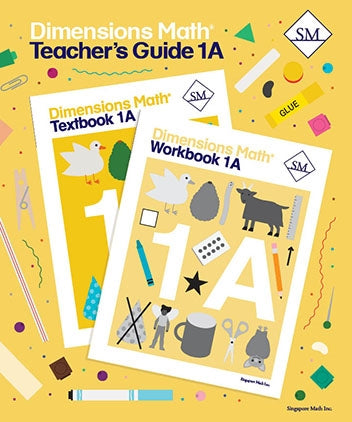# Dimensions Math Teacher's Guide 1A

Dimensions Math® Teacher's Guide 1A

Chapter 1: Numbers to 10

Teaching Notes
Chapter Opener
Lesson 1: Numbers to 10
Lesson 2: The Number 0
Lesson 3: Order Numbers
Lesson 4: Compare Numbers
Lesson 5: Practice
Workbook Solutions

Chapter 2: Number Bonds

Teaching Notes
Chapter Opener
Lesson 1: Make 6
Lesson 2: Make 7
Lesson 3: Make 8
Lesson 4: Make 9
Lesson 5: Make 10 — Part 1
Lesson 6: Make 10 — Part 2
Lesson 7: Practice
Workbook Solutions

Teaching Notes
Chapter Opener
Lesson 1: Addition as Putting Together
Lesson 4: Addition with Number Bonds
Lesson 5: Addition by Counting On
Lesson 8: Practice
Workbook Solutions

Chapter 4: Subtraction

Teaching Notes
Chapter Opener
Lesson 1: Subtraction as Taking Away
Lesson 2: Subtraction as Taking Apart
Lesson 3: Subtraction by Counting Back
Lesson 4: Subtraction with 0
Lesson 5: Make Subtraction Stories
Lesson 6: Subtraction with Number Bonds
Lesson 8: Make Addition and Subtraction Story Problems
Lesson 9: Subtraction Facts
Lesson 10: Practice
Review 1
Workbook Solutions

Chapter 5: Numbers to 20

Teaching Notes
Chapter Opener
Lesson 1: Numbers to 20
Lesson 2: Add or Subtract Tens or Ones
Lesson 3: Order Numbers to 20
Lesson 4: Compare Numbers to 20
Lesson 6: Subtraction
Lesson 7: Practice
Workbook Solutions

Teaching Notes
Chapter Opener
Lesson 1: Add by Making 10 — Part 1
Lesson 2: Add by Making 10 — Part 2
Lesson 3: Add by Making 10 — Part 3
Lesson 4: Addition Facts to 20
Lesson 5: Practice
Workbook Solutions

Chapter 7: Subtraction Within 20

Teaching Notes
Chapter Opener
Lesson 1: Subtract from 10 — Part 1
Lesson 2: Subtract from 10 — Part 2
Lesson 3: Subtract the Ones First
Lesson 4: Word Problems
Lesson 5: Subtraction Facts Within 20
Lesson 6: Practice
Workbook Solutions

Chapter 8: Shapes

Teaching Notes
Chapter Opener
Lesson 1: Solid and Flat Shapes
Lesson 2: Grouping Shapes
Lesson 3: Making Shapes
Lesson 4: Practice
Workbook Solutions

Chapter 9: Ordinal Numbers

Teaching Notes
Chapter Opener
Lesson 1: Naming Positions
Lesson 2: Word Problems
Lesson 3: Practice
Review 2
Workbook Solutions

Blackline Masters for 1A

Dimensions Math® PreK-5 series features the progression, rigor, and pacing that define Singapore math. Throughout the series, five characters offer students suggestions on how to think about problems. They remind students of strategies they’ve learned and point out important information that encourages them to come up with their own solutions.

Textbook lessons begin with a task that allows students to apply their previous knowledge and learn through discussion. Once students have mastered a concept with the use of concrete and pictorial aids, they are ready to take on more abstract mathematical problem sets. They reach fluency by collecting various strategies along the way and applying them to new problems. Word problems give students a sense of math in real-world contexts.

Workbooks offer independent practice that follows a careful progression of exercise variation. Each textbook lesson includes a corresponding workbook exercise that starts with pictorial representation and progresses to more challenging abstract problems. Workbooks for PreK-2 are perforated.

Teacher’s Guides include lesson plans, mathematical background, games, helpful suggestions, and comprehensive resources for daily lessons. Lessons are laid out clearly and activities are designed for the whole class, small groups, and extension.

Textbooks and Workbooks do not include answer keys. Answers are in Teacher's Guides.
ISBN  9781947226326